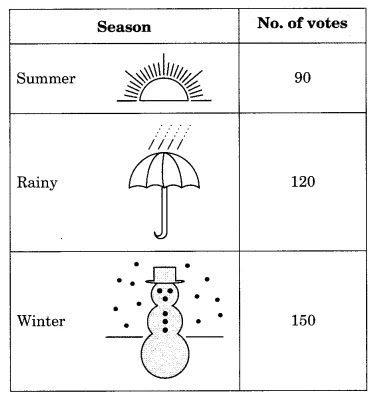NCERT Solutions for Class 8 Maths Chapter 5 Data Handling Ex 5.2

# NCERT Solutions for Class 8 Maths Chapter 5 Data Handling Ex 5.2

## NCERT Solutions for Class 8 Maths Chapter 5 Data Handling Ex 5.2

NCERT Solutions for Class 8 Maths Chapter 5 Data Handling Ex 5.2 are the part of NCERT Solutions for Class 8 Maths. Here you can find the NCERT Solutions for Class 8 Maths Chapter 5 Data Handling Ex 5.2.

### Ex 5.2 Class 8 Maths Question 1.

A survey was made to find the type of music that a certain group of young people liked in a city. The following pie chart shows the findings of this survey.
From this pie chart answer the following:
(i) If 20 people liked classical music, how many young people were surveyed?
(ii) Which type of music is liked by the maximum number of people?
(iii) If a cassette company were to make 1000 CDs, how many of each type would they make?

Solution:
(i) Number of young people who were surveyed = (100 × 20)/10 = 200 people.
(ii) Light music is liked by the maximum number of people, i.e., 40% of people.
(iii) Total number of CDs = 1000
Number of CDs of classical music = (10 × 1000)/100 = 100
Number of CDs of semi-classical music = (20 × 1000)/100 = 200
Number of CDs of light music = (40 × 1000)/100 = 400
Number of CDs of folk music = (30 × 1000)/100 = 300

### Ex 5.2 Class 8 Maths Question 2.

A group of 360 people were asked to vote for their favourite season from the three seasons rainy, winter and summer.(i) Which season got the most votes?
(ii) Find the central angle of each sector.
(iii) Draw a pie chart to show this information.

Solution:
(i) Winter season got the most votes, i.e., 150.

### Ex 5.2 Class 8 Maths Question 3.

Draw a pie chart showing the following information. The table shows the colours preferred by a group of people.### Ex 5.2 Class 8 Maths Question 4.

The following pie chart gives the marks scored in an examination by a student in Hindi, English, Mathematics, Social Science and Science. If the total marks obtained by the students were 540, answer the following questions.
(i) In which subject did the student score 105 marks?
(Hint: for 540 marks, the central angle = 360°. So, for 105 marks, what is the central angle?)
(ii) How many more marks were obtained by the student in Mathematics than in Hindi?
(iii) Examine whether the sum of the marks obtained in Social Science and Mathematics is more than that in Science and Hindi.
(Hint: Just study the central angles).

Solution:

 Subjects Central angles Mathematics 90° English 55° Hindi 70° Science 80° S. Science 65°

(i) For 540 marks, the central angle = 360°
For 105 marks, the central angle = (360 × 105)/540 = 70°
Corresponding subject = Hindi
(ii) Marks obtained in Mathematics = (90 × 540)/360 = 135
More marks obtained by student in Mathematics than in Hindi = 135 – 105 = 30
(iii) Central angle of Social Science + Mathematics = 65° + 90° = 155°
Central angle of Science + Hindi = 80° + 70° = 150°

Therefore, the sum of the marks obtained in Social Science and Mathematics is more than that in Science and Hindi.

### Ex 5.2 Class 8 Maths Question 5.

The number of students in a hostel, speaking different languages is given below. Display the data in a pie chart.

Solution:

You can also like these:

NCERT Solutions for Maths Class 9

NCERT Solutions for Maths Class 10

NCERT Solutions for Maths Class 11

NCERT Solutions for Maths Class 12